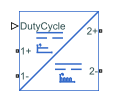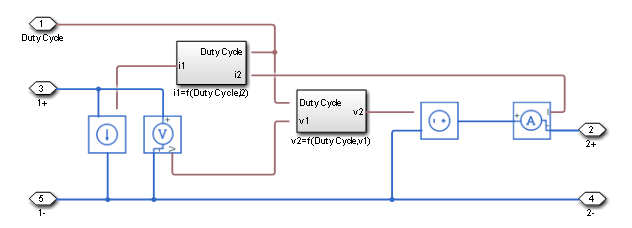# Average-Value Chopper

Average-value chopper

• Library:
• Simscape / Electrical / Semiconductors & Converters / Converters

•## Description

The Average-Value Chopper block represents a controlled average-value chopper. Use the duty cycle input to convert the electrical energy between the two sides. The figure shows the equivalent circuit for the block.### Equations

The input current and output voltage depend on the chopper class that you specify.

Voltage and Current Equations

Chopper ClassQuadrantsOutput Voltage, ${v}_{2}$Input Current, ${i}_{1}$
${i}_{2}<0$${i}_{2}=0$${i}_{2}>0$${i}_{2}<0$${i}_{2}=0$${i}_{2}>0$
A1st${v}_{2}={v}_{1}$${v}_{2}=DutyCycle\cdot {v}_{1}$${i}_{1}={i}_{2}$${i}_{1}=DutyCycle\cdot {i}_{2}$
B2nd${v}_{2}=\left(1-DutyCycle\right)\cdot {v}_{1}$${v}_{2}=0$${i}_{1}=\left(1-DutyCycle\right)\cdot {i}_{2}$${i}_{1}=0$
C1st and 2nd${v}_{2}=DutyCycle\cdot {v}_{1}$${i}_{1}=DutyCycle\cdot {i}_{2}$
D1st and 4th${v}_{2}={v}_{1}$${v}_{2}=\left(2\cdot DutyCycle-1\right)\cdot {v}_{1}$${i}_{1}={i}_{2}$${i}_{1}=\left(2\cdot DutyCycle-1\right)\cdot {i}_{2}$
EFour${v}_{2}=\left(2\cdot DutyCycle-1\right)\cdot {v}_{1}$${i}_{1}=\left(2\cdot DutyCycle-1\right)\cdot {i}_{2}$

### Limitations and Assumptions

• Input voltage, v1 is positive.

• Power losses are neglected.

• The Average-Value Chopper block represents an ideal implementation of a controlled average-value chopper with no dynamics, and it is suitable for low fidelity simulations. For higher fidelity models, use the One-Quadrant Chopper, Two-Quadrant Chopper, or Four-Quadrant Chopper blocks.

## Ports

### Conserving

expand all

Electrical conserving port associated with the duty cycle.

Data Types: `double`

Electrical conserving port associated with the positive terminal of the first DC voltage.

Data Types: `double`

Electrical conserving port associated with the negative terminal of the first DC voltage.

Data Types: `double`

Electrical conserving port associated with the positive terminal of the second DC voltage.

Data Types: `double`

Electrical conserving port associated with the negative terminal of the second DC voltage.

Data Types: `double`

## Parameters

expand all

Chopper class.

Specify the parameterization of the converter efficiency. If you select `Tabulated`, conduction losses will depend on the provided output current.

Efficiency of the converter, in percentage.

#### Dependencies

To enable this parameter, set Converter efficiency to `Constant`.

Vector of output currents.

#### Dependencies

To enable this parameter, set Converter efficiency to `Tabulated`.

Vector of efficiencies for each output current specified in Output current vector, in percentage. This parameter must have the same size of Output current vector.

#### Dependencies

To enable this parameter, set Converter efficiency to `Tabulated`.

 Trzynadlowski, A. M. Introduction to Modern Power Electronics. 2nd Ed. Hoboken, NJ: John Wiley & Sons Inc., 2010.